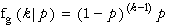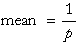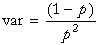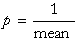# DPL 9 Help

Geometric Distribution

#Geometric Distribution

The Geometric Distribution is the discrete version of the Exponential Distribution. It has the same property as the Exponential Distribution in that conditional distributions are also geometric. The Geometric Distribution represents how long (in terms of the number of failures) one has to wait for a success. For example, each time you play a lottery there is the same probability of winning. Therefore, the number of times you play until your first win has a geometric distribution. One application of the Geometric Distribution is in Markov chain models, particularly meteorological models of weather cycles and amounts of precipitation. In failure analysis the Geometric Distribution represents the number of trials required to obtain the first failure, assuming the probability of failure is constant for each trial.

The Geometric Distribution represents the probability of getting a head for the first time on the kth flip of a biased coin. The probability of a head on any flip is p, the distribution's only parameter. The distribution is:where k=1,2,... and 0<p<1.

The equations for the mean and variance are:The equation for the parameter is:The mean must be greater than one.

Versions: DPL Professional, DPL Enterprise, DPL Portfolio